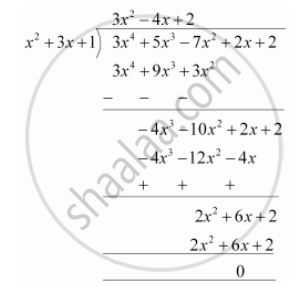# Check whether the first polynomial is a factor of the second polynomial by dividing the second polynomial by the first polynomial  x^2 + 3x + 1, 3x^4 + 5x^3 – 7x^2 + 2x + 2 - Mathematics

Check whether the first polynomial is a factor of the second polynomial by dividing the second polynomial by the first polynomial

x2 + 3x + 1, 3x4 + 5x3 – 7x2 + 2x + 2

#### Solution

x2 + 3x + 1, 3x4 + 5x3 – 7x2 + 2x + 2Since the remainder is 0

hence x+ 3x +1 is a factor of 3x4 + 5x3 - 7x2 + 2x + 2

Concept: Division Algorithm for Polynomials
Is there an error in this question or solution?
Chapter 2: Polynomials - Exercise 2.3 [Page 36]

#### APPEARS IN

NCERT Class 10 Maths
Chapter 2 Polynomials
Exercise 2.3 | Q 2.2 | Page 36

Share